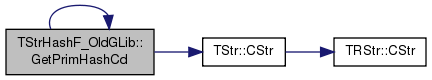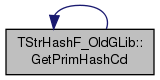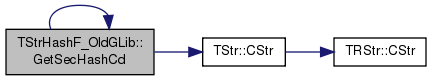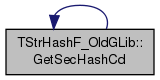SNAP Library 6.0, Developer Reference  2020-12-09 16:24:20 SNAP, a general purpose, high performance system for analysis and manipulation of large networks
TStrHashF_OldGLib Class Reference

`#include <hash.h>`

## Static Public Member Functions

static int GetPrimHashCd (const char *p)

static int GetSecHashCd (const char *p)

static int GetPrimHashCd (const TStr &s)

static int GetSecHashCd (const TStr &s)

## Detailed Description

Definition at line 1218 of file hash.h.

## Member Function Documentation

 static int TStrHashF_OldGLib::GetPrimHashCd ( const char * p )
inlinestatic

Definition at line 1220 of file hash.h.

1220  {
1221  const int MulBy = 16; // even older version used MulBy=2
1222  int HashCd = 0;
1223  while (*p) { HashCd = (MulBy * HashCd) + *p++; HashCd &= 0x0FFFFFFF; }
1224  return HashCd; }
 static int TStrHashF_OldGLib::GetPrimHashCd ( const TStr & s )
inlinestatic

Definition at line 1230 of file hash.h.

References TStr::CStr(), and GetPrimHashCd().

Referenced by GetPrimHashCd().

1230 { return GetPrimHashCd(s.CStr()); }
static int GetPrimHashCd(const char *p)
Definition: hash.h:1220
char * CStr()
Definition: dt.h:479

Here is the call graph for this function:Here is the caller graph for this function:static int TStrHashF_OldGLib::GetSecHashCd ( const char * p )
inlinestatic

Definition at line 1225 of file hash.h.

1225  {
1226  const int MulBy = 16; // even older version used MulBy=2
1227  int HashCd = 0;
1228  while (*p) { HashCd = (MulBy * HashCd) ^ *p++; HashCd &= 0x0FFFFFFF; }
1229  return HashCd; }
 static int TStrHashF_OldGLib::GetSecHashCd ( const TStr & s )
inlinestatic

Definition at line 1231 of file hash.h.

References TStr::CStr(), and GetSecHashCd().

Referenced by GetSecHashCd().

1231 { return GetSecHashCd(s.CStr()); }
char * CStr()
Definition: dt.h:479
static int GetSecHashCd(const char *p)
Definition: hash.h:1225

Here is the call graph for this function:Here is the caller graph for this function:The documentation for this class was generated from the following file: